### Home > CALC > Chapter 11 > Lesson 11.1.2 > Problem11-22

11-22.
1. Examine the integrals below. Consider the multiple tools available for integrating and use the best strategy. After evaluating each integral, write a short description of your method. Homework Help ✎

1.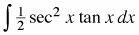2.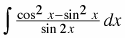3.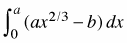4.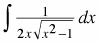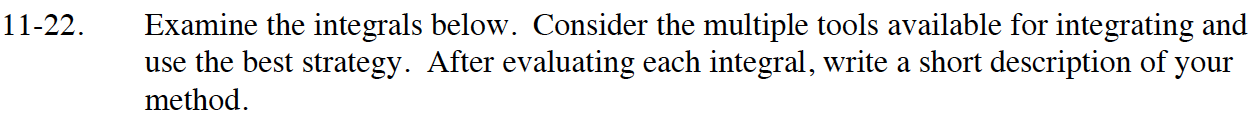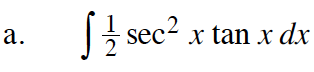Use u-substitution. Let u = sec(x).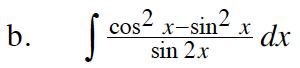cos2(x) – sin2(x) = cos(2x)

Use u-substitution. Let u = sin(2x).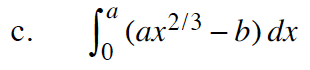Note: Given dx, x is the only variable.

$\left.=\frac{3}{5}ax^{5/3}-bx\right|_0^a$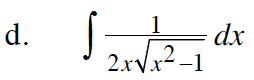The integrand is a multiple of an inverse trigonometric function. Which one?